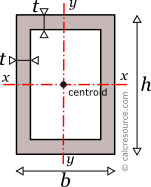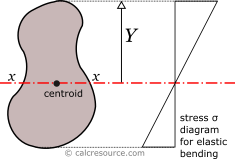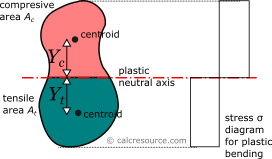Jump to
Table of Contents
Share this

## Properties of Rectangular Hollow Cross-Section

This tool calculates the properties of a rectangular hollow cross-section (also called tubular). Enter the shape dimensions b, h and t below. The calculated results will have the same units as your input. Please use consistent units for any input.

 b = h = t = Geometric properties: Area = Outer Per. = Inner Per. = Properties related to x-x axis: Ix = Sx = Zx = Rgx = Properties related to y-y axis: Iy = Sy = Zy = Rgy = Other properties: Iz =Table of Contents
Share this

## Definitions

### Geometry

The area A, the outer perimeter Poutand inner perimeter Pinof a rectangular hollow cross-section, can be found with the next formulas:

where b, h the external sides of the section and t the thickness of the walls.

### Moment of Inertia

The moment of inertia (second moment of area) of a rectangular hollow section, in respect to an axis x-x passing through its centroid, parallel to its base b, is given by the following expression:

where b is the base width, and specifically the dimension parallel to the axis, and h is the height (more specifically, the dimension perpendicular to the axis), , and t the thickness of the walls.

Similarly, the moment of inertia (second moment of area) of a rectangle with respect to a centroidal axis y-y perpendicular to its base, can be found, by alternating dimensions b and h, in the last equation:

The moment of inertia (second moment or area) is used in beam theory to describe the rigidity of a beam against flexure. The bending moment M applied to a cross-section is related with its moment of inertia with the following equation:

where E is the Young's modulus, a property of the material, and κ the curvature of the beam due to the applied load. Therefore, it can be seen from the former equation, that when a certain bending moment M is applied to a beam cross-section, the developed curvature is reversely proportional to the moment of inertia I.

The polar moment of inertia, describes the rigidity of a cross-section against torsional moment, likewise the planar moments of inertia described above, are related to flexural bending. The calculation of the polar moment of inertia Izabout an axis z-z (perpendicular to the section), can be done with the Perpendicular Axes Theorem:

where the Ixand Iyare the moments of inertia about axes x-x and y-y that are mutually perpendicular with z-z and meet at a common origin.

The dimensions of moment of inertia are .

### Elastic section modulus

The elastic section modulus Sxof any cross section about axis x-x (centroidal), describes the response of the section under elastic flexural bending. It is defined as:

where Ixthe moment of inertia of the section about x-x axis and Y the distance from centroid of a section point (aka fiber, typically the most distant one), measured perpendicularly to x-x axis. If a cross-section is symmetric (like the section in this page) about an axis (e.g. x-x) and its dimension perpendicular to this axis is h, then Y=h/2 and the above formula becomes:

Similarly, for the section modulus Sy, relative to y-y axis, which happens to be symmetry axis too, the above definitions are written as:If a bending moment Mxis applied on axis x-x, the section will respond with normal stresses, varying linearly with the distance from the neutral axis (which under elastic regime coincides to the centroidal x-x axis). Along neutral axis the stresses are zero. Absolute maximum σ will occur at the most distant fiber, with magnitude given by the formula:

From the last equation, the section modulus can be considered for flexural bending, a property analogous to cross-sectional A, for axial loading. For the latter, the normal stress is F/A.

The dimensions of section modulus are .

### Plastic section modulus

The plastic section modulus is similar to the elastic one, but defined with the assumption of full plastic yielding of the cross section due to flexural bending. In that case the whole section is divided in two parts, one in tension and one in compression, each under uniform stress field. For materials with equal tensile and compressive yield stresses, this leads to the division of the section into two equal areas, Atin tension and Acin compression, separated by the neutral axis. The axis is called plastic neutral axis, and for non-symmetric sections, isn't the same with the elastic neutral axis (which again is the centroidal one). The plastic section modulus is given by the general formula:

where Ycthe distance of the centroid of the compressive area Acfrom the plastic neutral axis and Ytthe respective distance of the centroid of the tensile area At. For the case of a rectangular hollow cross-section, the plastic neutral axis passes through centroid, dividing the whole area into two equal parts. Taking advantage of symmetry, Yc=Ytand application of the above equation gives the following formula for the plastic section modulus, of the rectangular hollow cross section, under x-x bending:

where### Radius of gyration

Radius of gyration Rgof a cross-section, relative to an axis, is given by the formula:

where I the moment of inertia of the cross-section about the same axis and A its area. The dimensions of radius of gyration are . It describes how far from centroid the area is distributed. Small radius indicates a more compact cross-section. Circle is the shape with minimum radius of gyration, compared to any other section with the same area A.